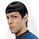1419 просмотров
Mr.Snake Chanell
Комментарий: Channel. Automatically shows the highs and lows, both local and global.
Knowing the previous high and at least you can predict the future price, the script indicates that for you.
You can change the weight and length and cross of your choice in the settings
```study("Mr.Snake Chanell", overlay=true)
Mr_Snake_Chanell = input("D")
Range = input(1)

SELL = security(tickerid, Mr_Snake_Chanell, highest(Range))

HI = plot(SELL, color=SELL!=SELL?na:black,linewidth=1 )
fill(HI, LO, color=white, transp=80)
Hcon = high >= SELL

plotshape(Hcon, style=shape.xcross, color=black, location=location.abovebar)
plotshape(Lcon, style=shape.xcross, color=black, location=location.belowbar)

Mr_Snake_Chanelli = input('W')
M_HIGH = security(tickerid, Mr_Snake_Chanelli, high)
M_LOW = security(tickerid, Mr_Snake_Chanelli, low)
plot(M_HIGH, color=M_HIGH != M_HIGH ?na:blue, style=line, linewidth=2)
plot(M_LOW, color=M_LOW != M_LOW ?na:blue, style=line, linewidth=2)
length = input(2)
hls = rma(hl2, length)
isRising = hls >= hls

```

## КомментарииChannel. Automatically shows the highs and lows, both local and global.
Knowing the previous high and at least you can predict the future price, the script indicates that for you.
You can change the weight and length and cross of your choice in the settings
Ответить